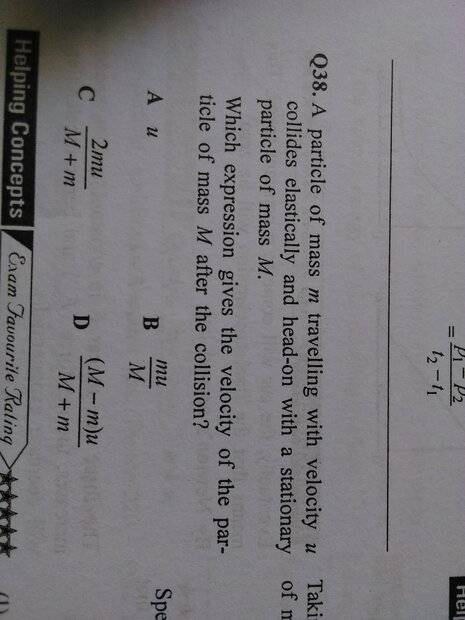# How to find resulting velocity in a perfectly elastic collision?

haha0p1
Homework Statement:
A particle of mass m travelling with velocity u collides elastically and head-on with a stationary particle of mass M. Which expression gives the velocity of the particle of mass M after the collision.
Relevant Equations:
Momentum=Mass×Velocity
Using principle of conservation of momentum:
m×u=m×v1 + M×v2
Where m=mass of moving particle in the beginning
u=Initial velocity of particle m
v1= final velocity of particle m
v2=velocity of object M
m×u-(mv1)=Mv2
(mu-mv1)÷M=v2
However, it is nowhere close to the correct answer. Kindly tell where I am going wrong in the calculation.Homework Helper
Gold Member
2022 Award
You should not have v1 in the answer since that is also unknown. You have not used that the collision is perfectly elastic.

haha0p1
You should not have v1 in the answer since that is also unknown. You have not used that the collision is perfectly elastic.
I have used V1 im the equation because the initial momentum is equal to final momentum and V1 is a part of the final momentum.

Homework Helper
Gold Member
2022 Award
I have used V1 im the equation because the initial momentum is equal to final momentum and V1 is a part of the final momentum.
There's nothing wrong with it as an equation, but it is not acceptable as an answer. Only m, M and u are allowed. To eliminate v1 you need another equation, the equation for energy conservation.

•MatinSAR
haha0p1
Using principle of conservation of momentum:
mu=(m×v-u)+M×v
mu-m(v-u)=M×v
-mv÷M=v

Note:
m=mass of the initially moving object
v=velocity of object woth mass M
x-u=Velocity of object m after the collision

I have used a different method but I am still getting a wrong answer.

•